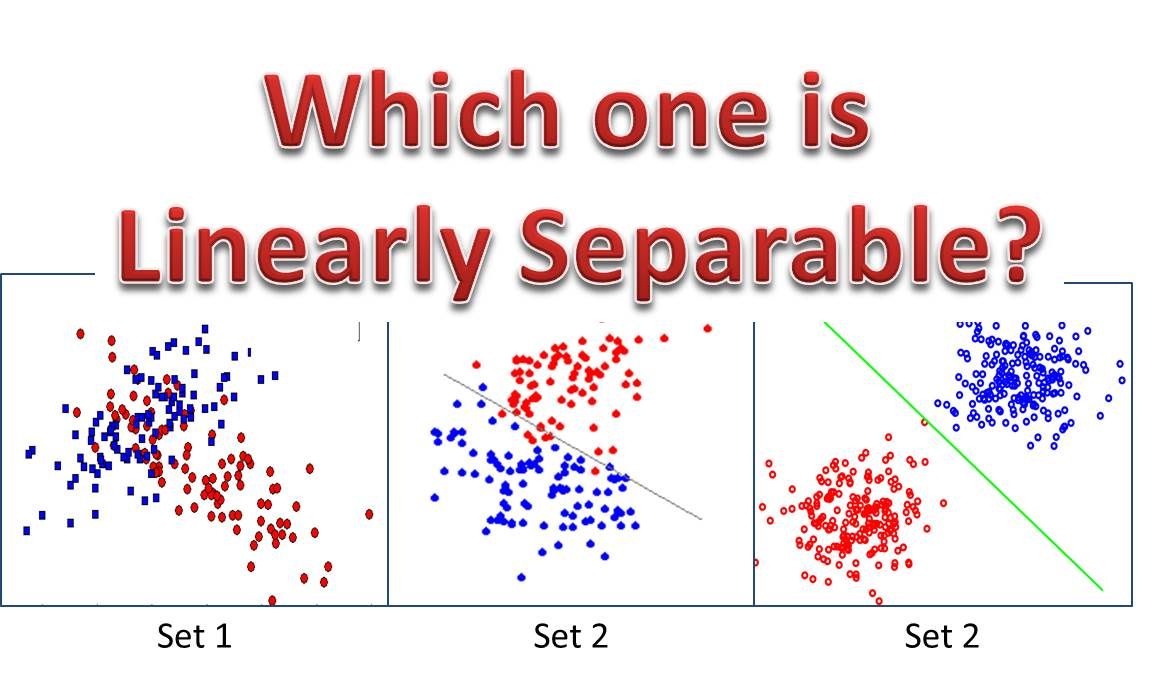# What is a Linear Separator? What is a Hyperplane? (Simple Explanation)Today, I would give a very simple explanation of the concept of linear separator and hyperplane.
This is would be a very basic and simple, easy to understand explanation, just to help you pass your exam or  a test.☺

Content

### 1. What is a Linear Separability?

To answer this question we need to first understand the concept of linear separability.
First, the concept of linear separation applies to a set of points.

The two sets of points are said to be linearly separable if a line can be drawn that separates the points such that a set points is on one side of the line and another set of points is on the other side of the line.

Figure 1: Can you figure it out?

Now take a look at Figure 1. Can figure out which set of points are linearly separable?
For sure, we could see that the answer is Set 3.

### 2. Formal Definition of Linear Separation

Assuming that the blue data sets represents set of point X0
The red data sets represents set of point X1

Then the two sets X0 and X1 are linearly separable if there exist n+1 real numbers:
w1, w2, …, wn, k

such that every point in X0  satisfies the relation

and every point in X1 satisfies the relation

### 3. What is a Linear Separator?

From what we’ve discussed so far, can you say what a Linear Separator is? It is simply the plane/line that separates the two sets of data!
(I must say, that formal definitions make simple concepts appear difficult)

But let’s take a formal definition
A linear separator is a a vector-threshold pair, (w, k) that satisfies the two relations given above

### 4. What is a Hyperplane?

As I mentioned, sometimes the terms used makes the concept appear complicated. The concepts of hyperplane is very simple: A hyperplane is just a plane that is one dimension less than the current space being considered.

Quiz 1: If a space is a 3-dimensional space, what would be the hyperplanes?